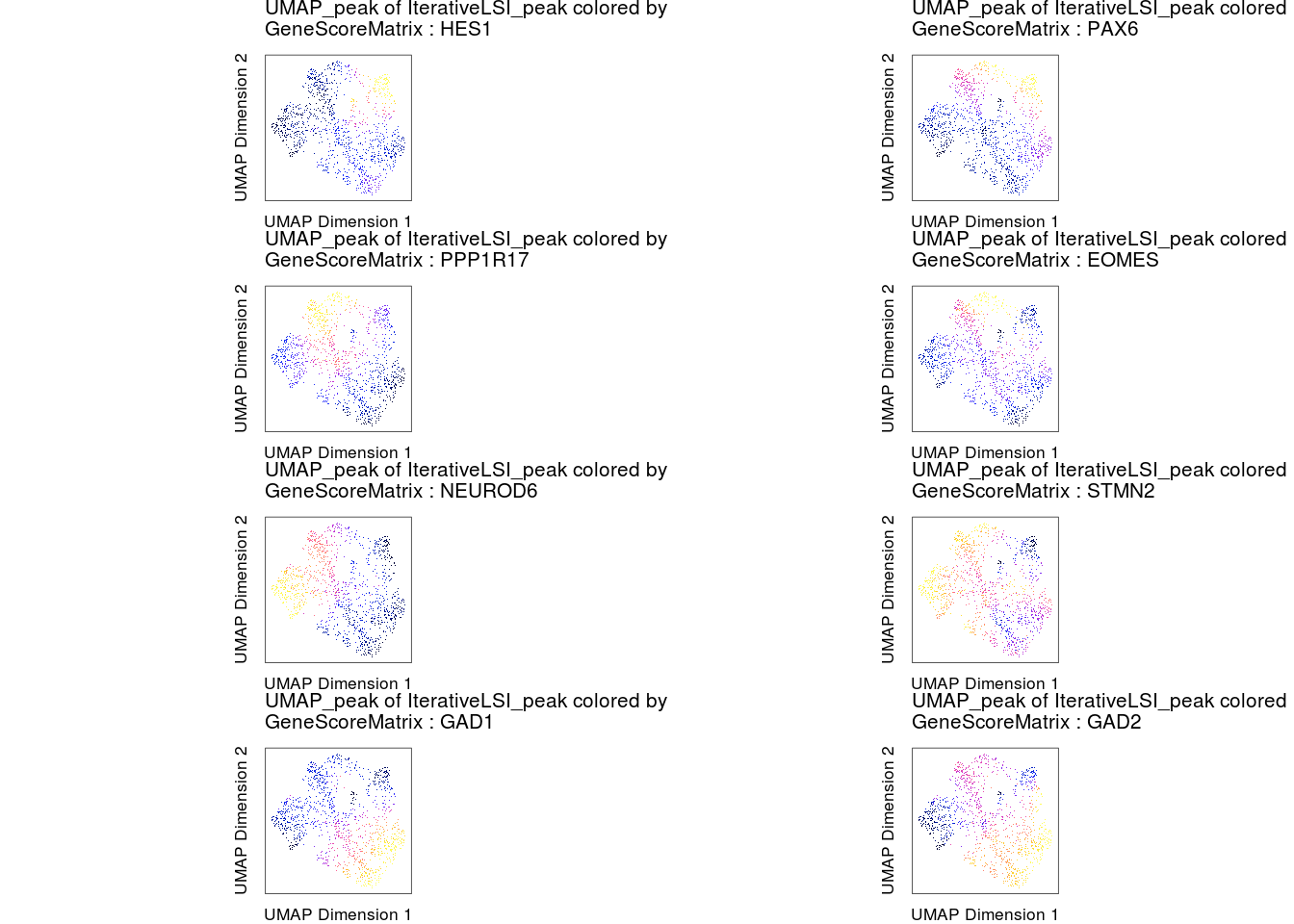# Chapter 15 Annotate by Gene Scores for Canonical Genes

``````library(ArchR)
set.seed(1)``````

## 15.1 Description

• Visualize gene scores for canonical marker genes to annotate

## 15.2 Set env and load arrow project

``````## Section: set default para
##################################################

##################################################

## Section: imputation
##################################################
proj <- addImputeWeights(proj, reducedDims = "IterativeLSI_peak")``````

## 15.3 Visualize gene scores

``````markerGenes <- c("HES1", "PAX6",
"PPP1R17", "EOMES",
"NEUROD6", "STMN2",

p <- plotEmbedding(
ArchRProj = proj,
colorBy = "GeneScoreMatrix",
name = markerGenes,
embedding = "UMAP_peak",
quantCut = c(0.01, 0.95),
size = 0.5,
imputeWeights = getImputeWeights(proj)
)``````
``## Getting ImputeWeights``
``````## ArchR logging to : ArchRLogs/ArchR-plotEmbedding-2aee3c8a3286-Date-2021-07-26_Time-21-01-43.log
## If there is an issue, please report to github with logFile!``````
``## Getting UMAP Embedding``
``## ColorBy = GeneScoreMatrix``
``## Getting Matrix Values...``
``## 2021-07-26 21:01:45 :``
``## ``
``## Imputing Matrix``
``## Using weights on disk``
``## 1 of 1``
``## Plotting Embedding``
``````## 1 2 3 4 5 6 7 8
## ArchR logging successful to : ArchRLogs/ArchR-plotEmbedding-2aee3c8a3286-Date-2021-07-26_Time-21-01-43.log``````
``````#Rearrange for grid plotting
p2 <- lapply(p, function(x) {
x + guides(color = FALSE, fill = FALSE) +
theme_ArchR(baseSize = 6.5) +
theme(plot.margin = unit(c(0, 0, 0, 0), "cm")) +
theme(
axis.text.x = element_blank(),
axis.ticks.x = element_blank(),
axis.text.y = element_blank(),
axis.ticks.y = element_blank()
)
})

do.call(cowplot::plot_grid, c(list(ncol = 2),p2))``````